# User:Tohline/Appendix/Ramblings/ConcentricEllipsodalCoordinates

(Difference between revisions)
 Revision as of 08:51, 26 October 2020 (view source)Tohline (Talk | contribs) (→Concentric Ellipsoidal (T6) Coordinates)← Older edit Revision as of 10:09, 27 October 2020 (view source)Tohline (Talk | contribs) (→Concentric Ellipsoidal (T6) Coordinates)Newer edit → Line 25: Line 25: - When $~\lambda_1 = a$, we obtain the standard definition of an ellipsoidal surface, it being understood that, $~q^2 = a^2/b^2$ and $~p^2 = a^2/c^2$.  (We will assume that $~a > b > c$, that is, $~p^2 > q^2 > 1$.)  What is the expression for the unit vector normal to the surface at $~(x, y, z)$ when written in terms of Cartesian unit vectors? + When $~\lambda_1 = a$, we obtain the standard definition of an ellipsoidal surface, it being understood that, $~q^2 = a^2/b^2$ and $~p^2 = a^2/c^2$.  (We will assume that $~a > b > c$, that is, $~p^2 > q^2 > 1$.) + + A vector, $~\bold{\hat{n}}$, that is normal to the $~\lambda_1$ = constant surface is given by the gradient of the function, + + +
+ $~F(x, y, z)$ + + $~\equiv$ + + $~(x^2 + q^2 y^2 + p^2 z^2)^{1 / 2} - \lambda_1 \, .$ +
+ + + + + + + In Cartesian coordinates, this means, + + +
+ $~\bold{\hat{n}}(x, y, z)$ + + $~=$ + + $~ + \hat\imath \biggl( \frac{\partial F}{\partial x} \biggr) + + \hat\jmath \biggl( \frac{\partial F}{\partial y} \biggr) + + \hat{k} \biggl( \frac{\partial F}{\partial z} \biggr) +$ +
+   + + $~=$ + + $~ + \hat\imath \biggl[ x(x^2 + q^2 y^2 + p^2 z^2)^{- 1 / 2} \biggr] + + \hat\jmath \biggl[ q^2y(x^2 + q^2 y^2 + p^2 z^2)^{- 1 / 2} \biggr] + + \hat\jmath \biggl[ p^2 z(x^2 + q^2 y^2 + p^2 z^2)^{- 1 / 2} \biggr] +$ +
+   + + $~=$ + + $~ + \hat\imath \biggl( \frac{x}{\lambda_1} \biggr) + + \hat\jmath \biggl( \frac{q^2y}{\lambda_1} \biggr) + + \hat\jmath \biggl(\frac{p^2 z}{\lambda_1} \biggr) \, , +$ +
+ + + + + + + + + + + + + + + + + + where it is understood that this expression is only to be evaluated at points, $~(x, y, z)$, that lie on the selected $~\lambda_1$ surface — that is, at points for which the function, $~F(x,y,z) = 0$.  The length of this normal vector is given by the expression, + + +
+ $~[ \bold{\hat{n}} \cdot \bold{\hat{n}} ]^{1 / 2}$ + + $~=$ + + $~ + \biggl[ \biggl( \frac{\partial F}{\partial x} \biggr)^2 + \biggl( \frac{\partial F}{\partial y} \biggr)^2 + \biggl( \frac{\partial F}{\partial z} \biggr)^2 \biggr]^{1 / 2} +$ +
+ + + + + + + + + The properly normalized + + Next, we appreciate that the vector that is normal to theWhat is the expression for the unit vector normal to the surface at $~(x, y, z)$ when written in terms of Cartesian unit vectors? Well, to start with we know that $~\lambda_1^2$ is constant across the entire surface, so at any point on this specified surface we must find, Well, to start with we know that $~\lambda_1^2$ is constant across the entire surface, so at any point on this specified surface we must find,

# Concentric Ellipsoidal (T6) Coordinates

## Background

Building on our general introduction to Direction Cosines in the context of orthogonal curvilinear coordinate systems, and on our previous development of T3 (concentric oblate-spheroidal) and T5 (concentric elliptic) coordinate systems, here we explore the creation of a concentric ellipsoidal (T6) coordinate system. This is motivated by our desire to construct a fully analytically prescribable model of a nonuniform-density ellipsoidal configuration that is an analog to Riemann S-Type ellipsoids.

## Orthogonal Coordinates

We start by defining a "radial" coordinate whose values identify various concentric ellipsoidal shells,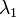$~\lambda_1$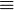$~\equiv$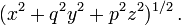$~(x^2 + q^2 y^2 + p^2 z^2)^{1 / 2} \, .$

When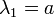$~\lambda_1 = a$, we obtain the standard definition of an ellipsoidal surface, it being understood that,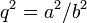$~q^2 = a^2/b^2$ and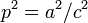$~p^2 = a^2/c^2$. (We will assume that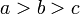$~a > b > c$, that is,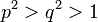$~p^2 > q^2 > 1$.)

A vector,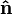$~\bold{\hat{n}}$, that is normal to the$~\lambda_1$ = constant surface is given by the gradient of the function,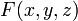$~F(x, y, z)$$~\equiv$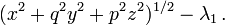$~(x^2 + q^2 y^2 + p^2 z^2)^{1 / 2} - \lambda_1 \, .$

In Cartesian coordinates, this means,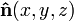$~\bold{\hat{n}}(x, y, z)$$~=$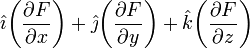$~ \hat\imath \biggl( \frac{\partial F}{\partial x} \biggr) + \hat\jmath \biggl( \frac{\partial F}{\partial y} \biggr) + \hat{k} \biggl( \frac{\partial F}{\partial z} \biggr)$$~=$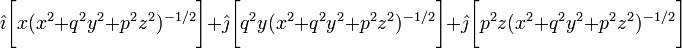$~ \hat\imath \biggl[ x(x^2 + q^2 y^2 + p^2 z^2)^{- 1 / 2} \biggr] + \hat\jmath \biggl[ q^2y(x^2 + q^2 y^2 + p^2 z^2)^{- 1 / 2} \biggr] + \hat\jmath \biggl[ p^2 z(x^2 + q^2 y^2 + p^2 z^2)^{- 1 / 2} \biggr]$$~=$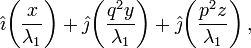$~ \hat\imath \biggl( \frac{x}{\lambda_1} \biggr) + \hat\jmath \biggl( \frac{q^2y}{\lambda_1} \biggr) + \hat\jmath \biggl(\frac{p^2 z}{\lambda_1} \biggr) \, ,$

where it is understood that this expression is only to be evaluated at points,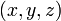$~(x, y, z)$, that lie on the selected$~\lambda_1$ surface — that is, at points for which the function,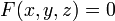$~F(x,y,z) = 0$. The length of this normal vector is given by the expression,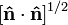$~[ \bold{\hat{n}} \cdot \bold{\hat{n}} ]^{1 / 2}$$~=$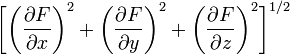$~ \biggl[ \biggl( \frac{\partial F}{\partial x} \biggr)^2 + \biggl( \frac{\partial F}{\partial y} \biggr)^2 + \biggl( \frac{\partial F}{\partial z} \biggr)^2 \biggr]^{1 / 2}$

The properly normalized

Next, we appreciate that the vector that is normal to theWhat is the expression for the unit vector normal to the surface at$~(x, y, z)$ when written in terms of Cartesian unit vectors?

Well, to start with we know that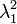$~\lambda_1^2$ is constant across the entire surface, so at any point on this specified surface we must find,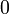$~0$$~=$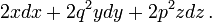$~2x dx + 2q^2y dy + 2p^2z dz \, .$